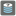• # help for len(denominations) != 3

## Question related to missionMaking Change

def checkio(price, denominations): temp = [] for i in range(0, price // denominations + 1): for j in range(0, price // denominations + 1): for k in range(0, price // denominations + 1): if price == denominations * i + denominations * j + denominations * k: temp.append(i+j+k) if not temp: return None else: return min(temp)

I don't know how can modify my codes if len(denominations) != 3.